From Tables and Spreadsheets to Data Cubes

• A data warehouse is based on a multidimensional data model which views data in the form of a data cube
• A data cube, such as sales, allows data to be modeled and viewed in multiple dimensions
• Dimension tables, such as item (item_name, brand, type), or time(day, week, month, quarter, year)
• Fact table contains measures (such as dollars_sold) and keys to each of the related dimension tables
• In data warehousing literature, an n-D base cube is called a base cuboid. The top most 0-D cuboid, which holds the highest-level of summarization, is called the apex cuboid. The lattice of cuboids forms a data cube.

Cube: A Lattice of Cuboids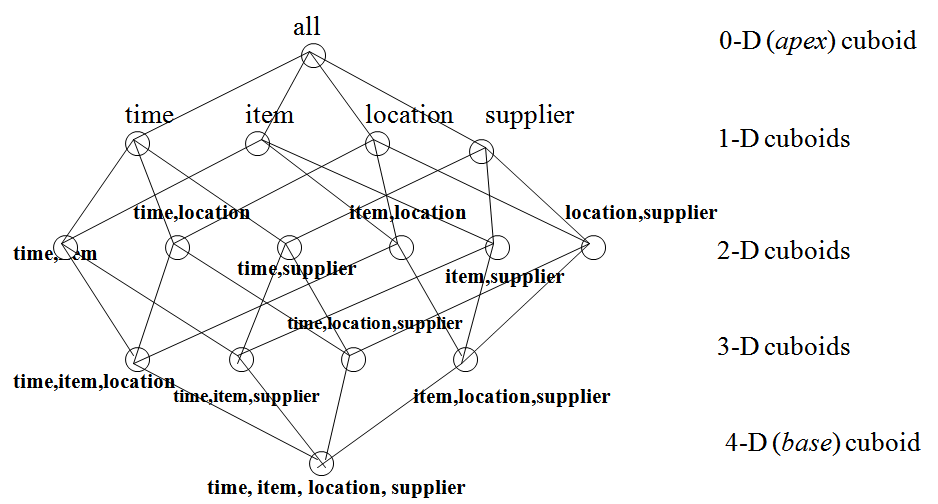Conceptual Modeling of Data Warehouses

• Modeling data warehouses: dimensions & measures
• Star schema: A fact table in the middle connected to a set of dimension tables
• Snowflake schema: A refinement of star schema where some dimensional hierarchy is normalized into a set of smaller dimension tables, forming a shape similar to snowflake
• Fact constellations: Multiple fact tables share dimension tables, viewed as a collection of stars, therefore called galaxy schema or fact constellation

Example of Star Schema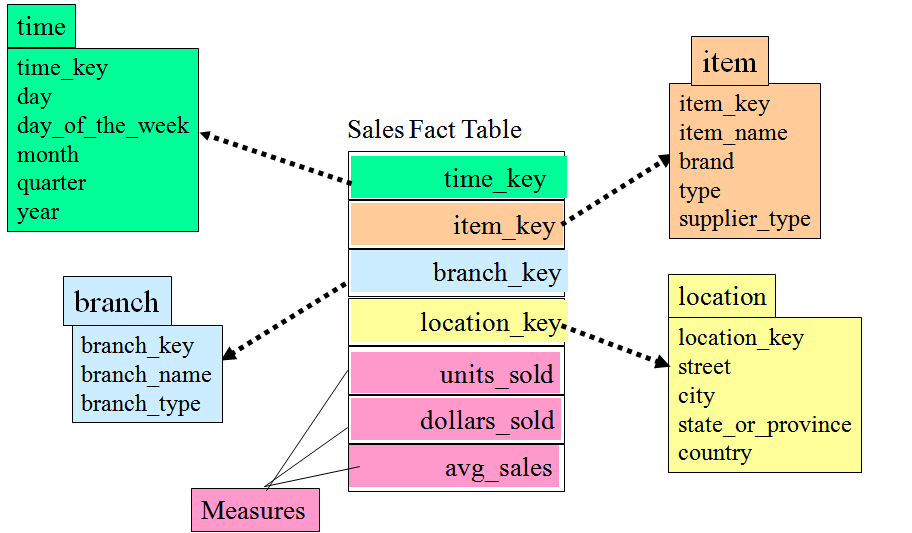Example of Snowflake Schema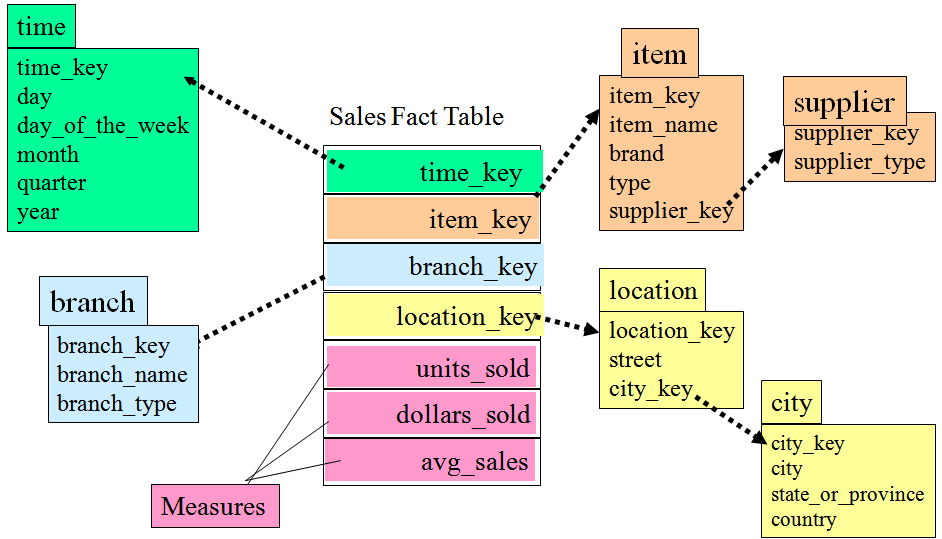Example of Fact Constellation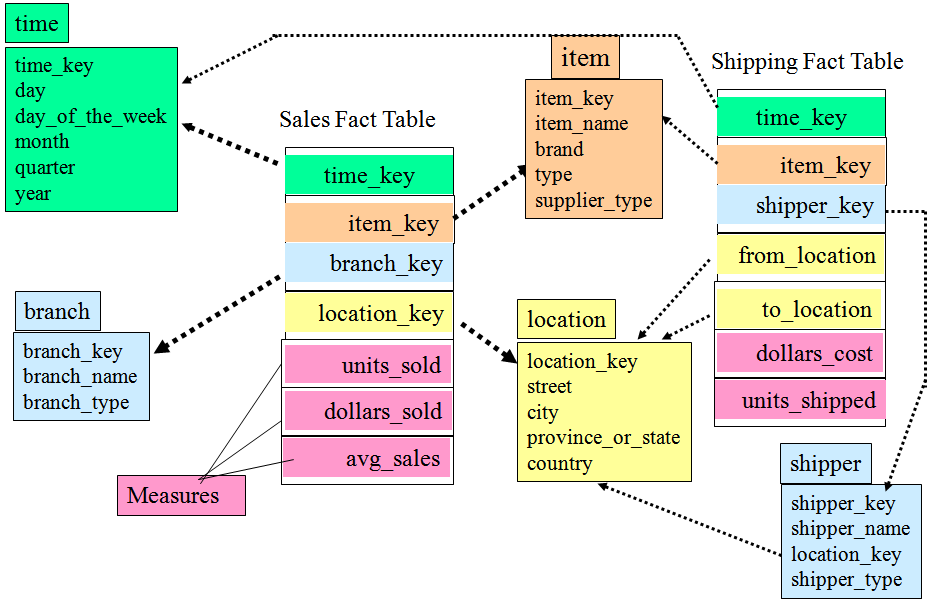Data Cube Measures: Three Categories

• Distributive: if the result derived by applying the function to n aggregate values is the same as that derived by applying the function on all the data without partitioning
• E.g., count(), sum(), min(), max()
• Algebraic: if it can be computed by an algebraic function with M arguments (where M is a bounded integer), each of which is obtained by applying a distributive aggregate function
• E.g., avg(), min_N(), standard_deviation()
• Holistic: if there is no constant bound on the storage size needed to describe a subaggregate.
• E.g., median(), mode(), rank()

View of Warehouses and HierarchiesMultidimensional Data

• Sales volume as a function of product, month, and region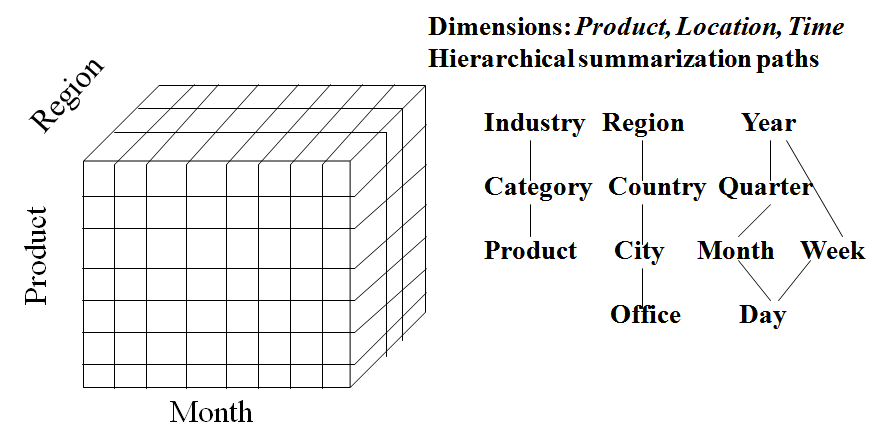A Sample Data CubeCuboids Corresponding to the CubeTypical OLAP Operations

• Roll up (drill-up): summarize data
• by climbing up hierarchy or by dimension reduction
• Drill down (roll down): reverse of roll-up
• from higher level summary to lower level summary or detailed data, or introducing new dimensions
• Slice and dice: project and select
• Pivot (rotate):
• reorient the cube, visualization, 3D to series of 2D planes
• Other operations
• drill across: involving (across) more than one fact table
• drill through: through the bottom level of the cube to its back-end relational tables (using SQL)

Typical OLAP Operations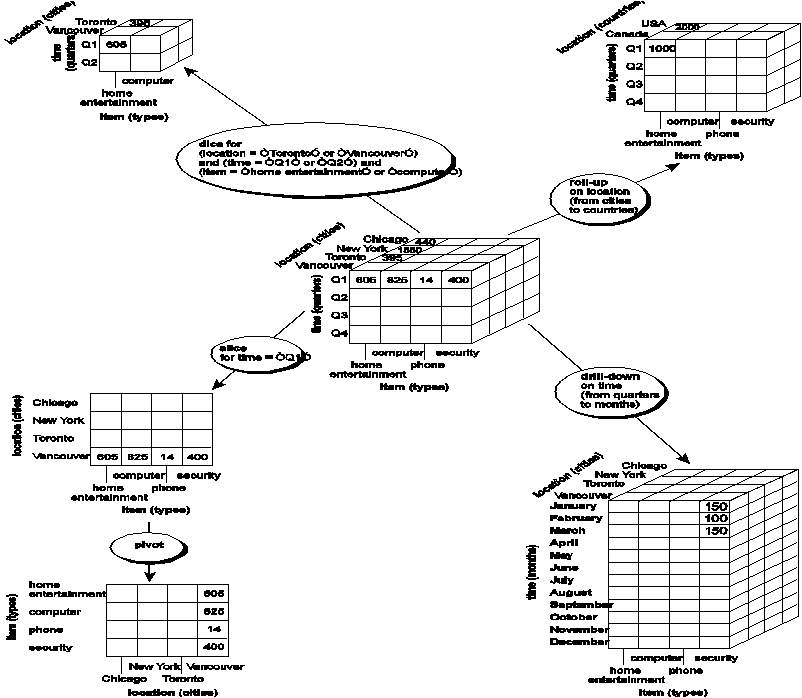A Star-Net Query Model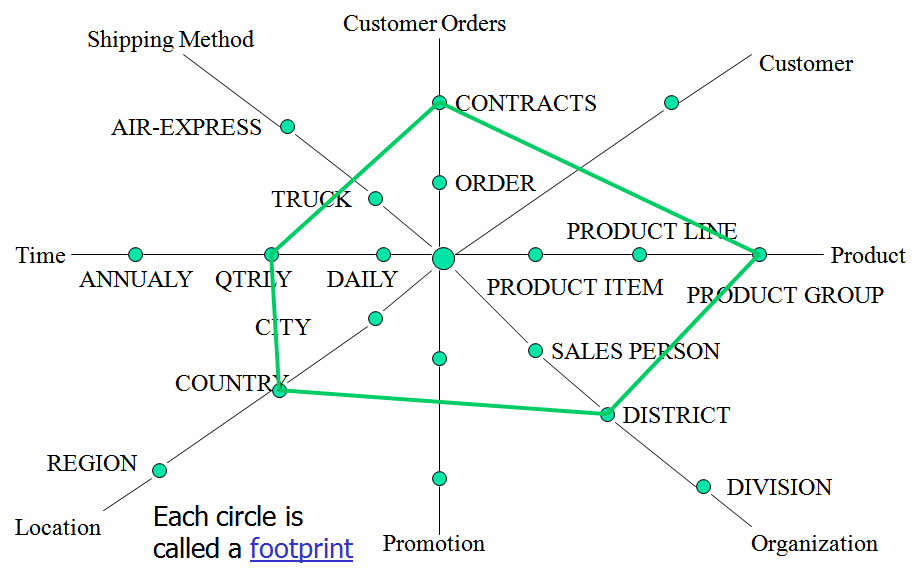Browsing a Data Cube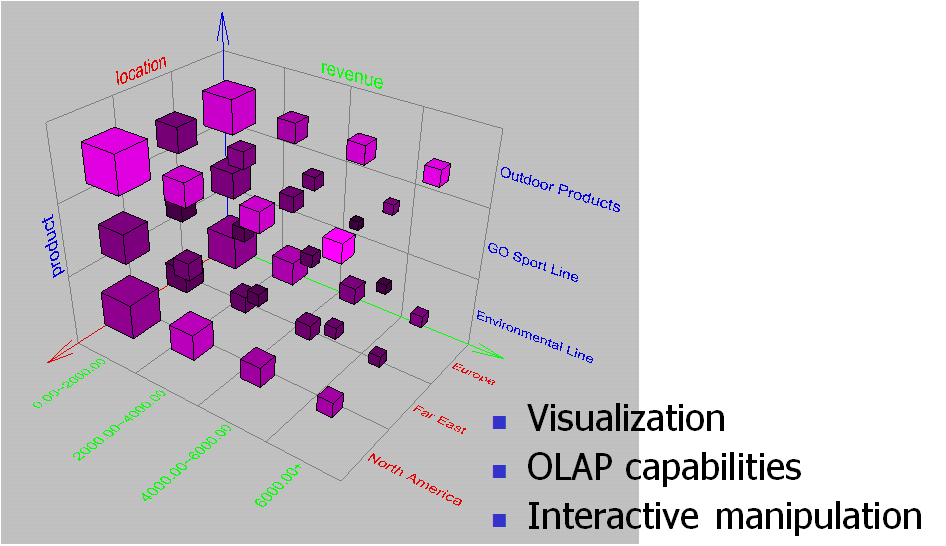Creator: sidraaslam

Contributors:
-